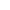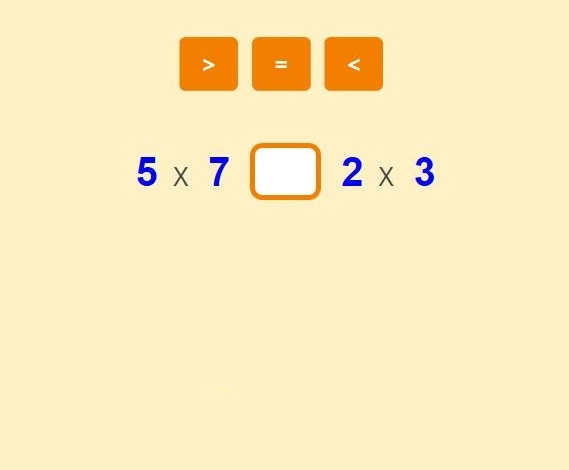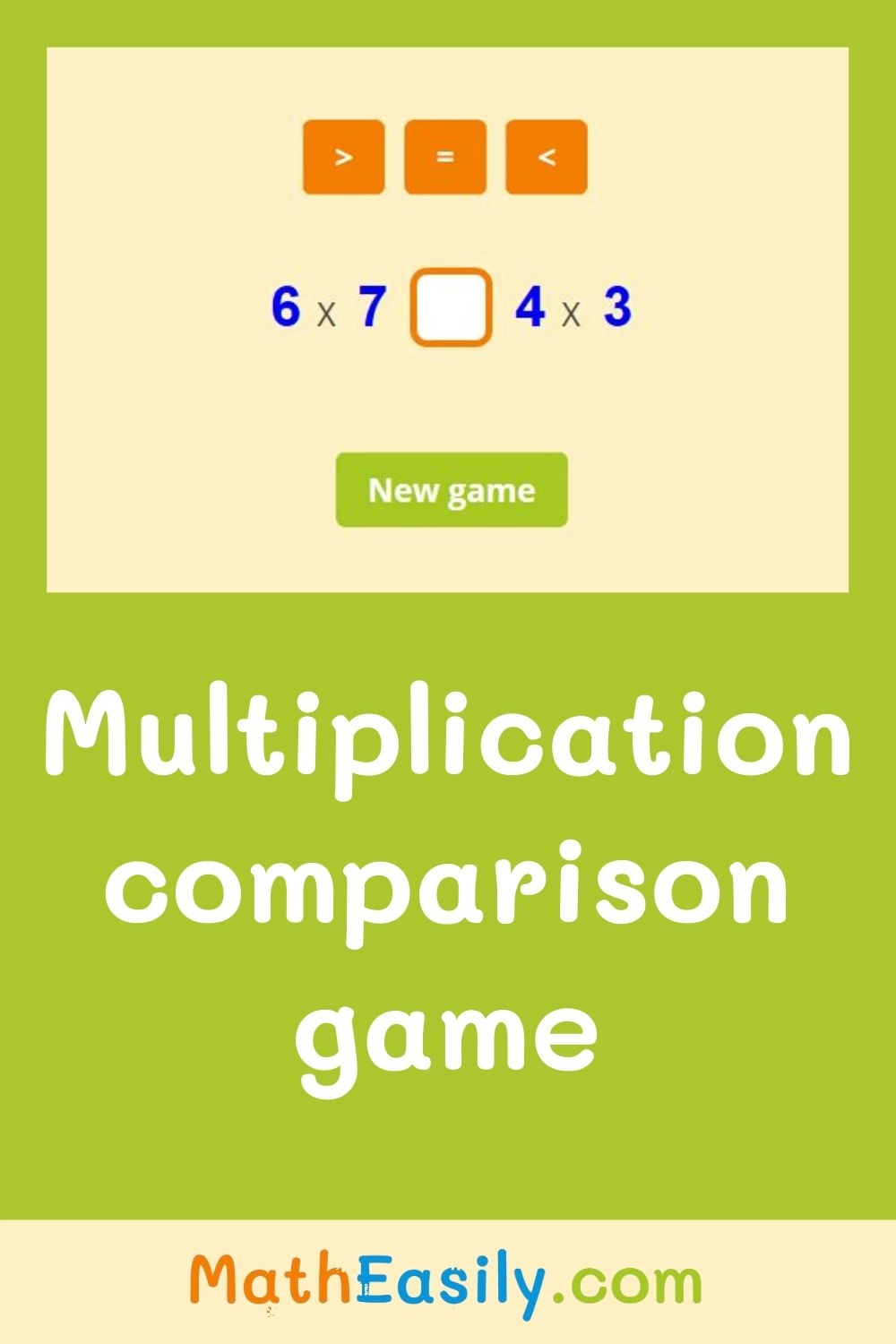﻿ Multiplication comparisons within 100 with answers

Page No. 1058

# Multiplication comparisons within 100

Multiplicative comparison 4th Grade. Multiplication comparisons within 100. multiplicative comparison games. multiplication comparisons worksheet.

X XNew game

Compare both results of the multicplication and click the correct symbol above.

## What is a multiplicative comparison?Multiplicative comparison means that you are comparing two things together that need to be multiplied. In following games and worksheets, first solve two multiplication problems and then compare both results of multiplication using the symbols for greater than, less than and equal.

## Why to play multiplicative comparison game?

This multiplication comparisons game teaches multiplication and comparing numbers at the same time. You can play this game online.

Do you want to try something more difficult? Then try this game: two digit multiplication.

Do you need to learn simple multiplication? Then try this one: single digit multiplication.

## Multiplication comparison: worksheets

Download our free printable multiplication comparisons worksheets in PDF. Fill in the blanks with the correct symbol. You can find all our multiplication worksheets here.

## Multiplication comparisons within 100 with answer key

This math game improves multiplication and comparison skills. Kids will love the challenge of this exercise that teaches them solving multiplication problems and then comparing the answers using the symbols for greater than, less than and equal.

Click the correct symbol above. If your result is OK, you will see "YES" sign.

If your answer is wrong, you will see "NO" sign. Try the other comparison symbol. Press the button "New game" and play another game.## Multiplicative comparison for 4th Grade Math Students

Our multiplicative comparison games teach multiplication and comparing numbers at the same time. Multiplication comparisons within 100 is determined for 4th Grade Math students. You can play this game online.

First, find the result of multiplication on the left side, remember it (or make note), then solve the multiplication on the right side. Now, compare both results. Click the right symbol above.

Do you need help with teaching multiplicative comparisons? Watch this tutorial on YouTube.